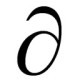## Rounding Up – Way Up

by

Ever heard of Dudeney numbers?  Neither had I, until yesterday, when I discovered them completely by accident while reading (Wikipedia, what else?) about narcissistic numbers.  A Dudeney number (named after famous English mathematician and puzzle author Henry Dudeney) is a number that is the cube of the sum of its digits.  For example,$4913 = 17^3 = (4+9+1+3)^3$

There are only six Dudeney numbers.  Neat numbers, but I was a little disappointed by that.  What to do next?

Generalize, of course!  Generalized Dudeney numbers (discussed here, but the link appears to be dead, so I used Google’s cached version) are numbers that are some power of the sum of their digits:$234256 = 22^4 = (2+3+4+2+5+6)^4$$12157665459056928801 \times 10^{20} = 90^{20} = (1+2+\cdots+0+0)^{20}$

The largest number on the above site is$547210^{25662}$, which has 147253 digits.  The site links to Wolfram Alpha to confirm this.  Here’s where it gets weird:How many digits is that?  About$10^6$?  About a million?  What kind of rounding is that?  It gets worse.  Try a number with just 100,002 digits (despite what Alpha says).  I think Alpha is a great tool, and I’ve had (far too much) fun playing with it, but I’m a tad disappointed (that’s twice in one post).  So, hey, get on that, Wolfram.

Tags: ,

### 4 Responses to “Rounding Up – Way Up”

1.theCountess Says:

This makes me sad. Wolfram Alpha is definitely my go-to for answers about life, the universe and everything. But how can I trust Alpha again if it thinks 100,001 is closer to one million than it is to 100,000?

Also, while looking around at Dudeney numbers I discovered all sorts of fun numbers (unfortunately, there are no actual “fun numbers”) like happy numbers, fortunate numbers (not to be confused with lucky numbers according to wikipedia), powerful numbers (also called squarefull numbers, which isn’t even word in my opinion) and friendly numbers (whose equivalence classes are called clubs). My new life goal is to seek out strange properties of numbers and name them clever things…

•Batman Says:

I think my favorite name might be “Achilles number”: powerful, but not perfect.

Also, no discussion of friendly numbers would be complete without mentioning this xkcd comic.

2. Yeah that’s cool, but it doesn’t have any applications. « PI (Repeating) Says:

[…] I was reading about Dudeney numbers.  Dudeney numbers, according to Wikipedia, are positive integers that are perfect cubic such that […]

3.DaveK Says:

Obviously, Wolfram is an astrophysicist.